### Adversarial Perturbations of Opinion Dynamics in Networks

Jason Gaitonde, Jon Kleinberg, Éva Tardos

Cornell University

2020

presented by Albert M Orozco Camacho

# Motivation

Opinion dynamics has been previously studied within the mathematical social sciences literature; yet not much attention has been put to current social network studies.

On the other hand, perturbations that induce discord in social media are of important interest for current research in politics, sociology, economics, computer science, etc.

## Adversarial Attacks to Induce Discord

The authors present a formal study on the intuition that a (maliscious) actor attacks the overall opinion consensus of a network.

These perturbations will difuse via an opinion dynamics model.

## Problem

An adversarial agent targets a few individual opinions to difuse a sense of discord.

Identify the most vulverable structures within a network. Mitigate these attacks by insulating nodes.

# Definitions

## Matrices

Adjacency Matrix $$A_{i,j} = A_{j,i} := w(i,j)$$

Diagonal Matrix $$D_{i,i} = \sum_{j \in V} w(i,j)$$

Laplacian Matrix $$L := D - A$$

### Some Spectral Properties

$$\mathbf{x}^T L \mathbf{x} = \sum_{(i,j) \in E} w(i,j)(\mathbf{x}(i)-\mathbf{x}(j))^2$$

$$L = \sum_{i=1}^n \lambda_i\mathbf{v}_i\mathbf{v}_i^T$$

### Friedkin-Johnsen Dynamics

Given $G = (V, E, w)$ and an initial opinion vector $s \in \mathbb{R}^n$.

Start with $\mathbf{z}^{(0)} = \mathbf{s}$.

For each node $i \in V$, update $$\mathbf{z}^{(t+1)}(i) = \frac{\mathbf{s}(i) + \sum_{j \in V} w(i,j)\mathbf{z}^{(T)}(j)} {1 + \sum_{j \in V} w(i,j)}$$

### Friedkin-Johnsen Dynamics

Convergence will be reached and the limiting final opinion vector is given by $$\mathbf{z} = (I + L)^{-1}\mathbf{s}$$

### General Formulation

$$\max_{s \in \mathbb{R}^n: ||\mathbf{s}||_2 \leq R} \mathbf{s}^T (I + L)^{-1}f(L)(I + L)^{-1}\mathbf{s}$$

### Disagreement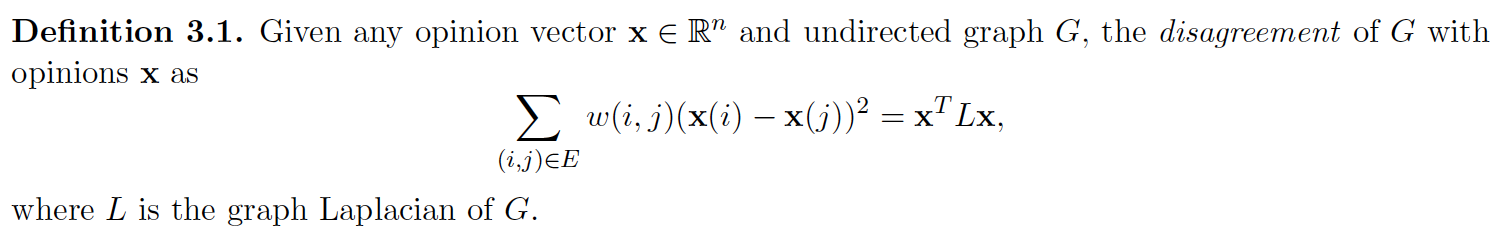### Optimizing Disagreement

CONCATENATION $$[h_v^{(1)},\ldots,h_v^{(k)}]$$

MAX-POOLING. Select the most informative layer for each feature coordinate.

LSTM-ATTENTION. Input $h_v^{(1)},\ldots,h_v^{(k)}$ into a bi-directional LSTM to generate forward and backward features $f_v^{(l)}$ and $b_v^{(l)}$ for each layer $l$; finally compute an attention score per each node by combining those for each layer.

### Polarization vs Disagreement

CONCATENATION $$[h_v^{(1)},\ldots,h_v^{(k)}]$$

MAX-POOLING. Select the most informative layer for each feature coordinate.

LSTM-ATTENTION. Input $h_v^{(1)},\ldots,h_v^{(k)}$ into a bi-directional LSTM to generate forward and backward features $f_v^{(l)}$ and $b_v^{(l)}$ for each layer $l$; finally compute an attention score per each node by combining those for each layer.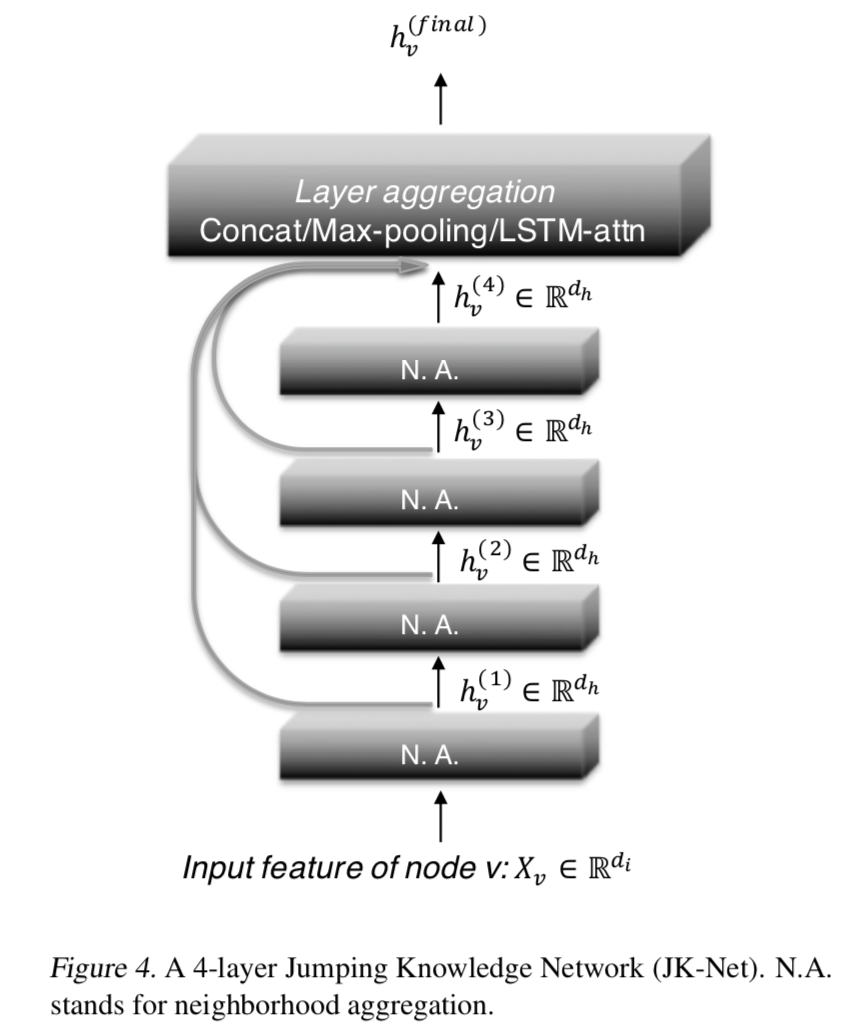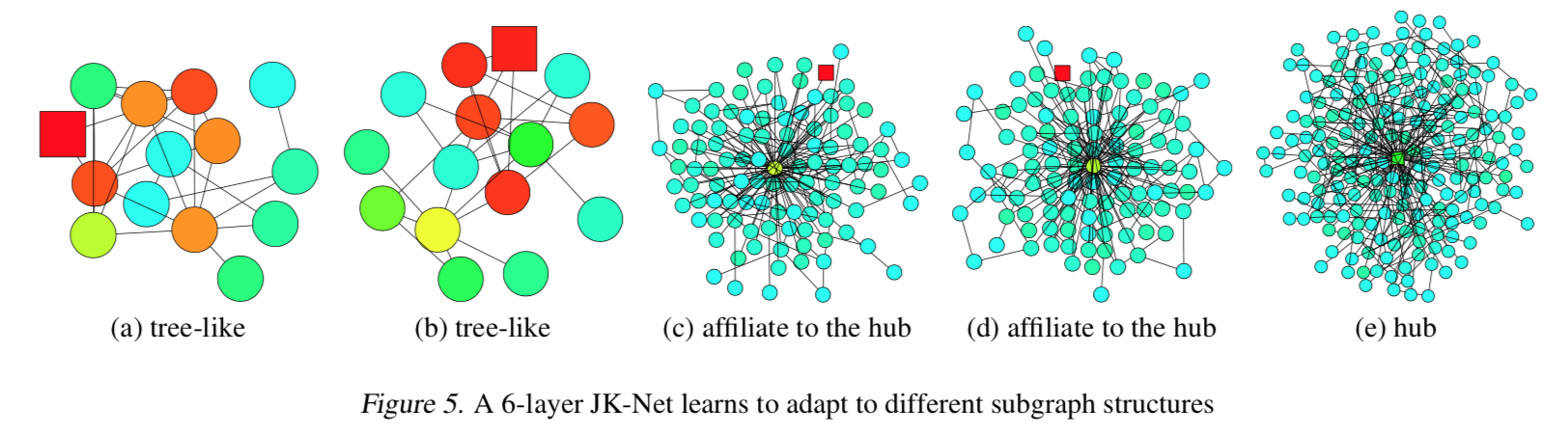### Proposition 1

Assume that paths of the same length in the computation graph are activated with the same probability.

The influence score $I(x, y)$ for any $x, y \in V$ under a $k$-layer JK-Net with layer-wise max-pooling is equivalent in expectation to a mixture of $0,\ldots,k$-step random walk distributions on $\tilde{G}$ at $y$ starting at $x$, the coefficients of which depend on the values of the layer features $h_x^{(l)}$.

# Mixed Graph Objectives

### Datasets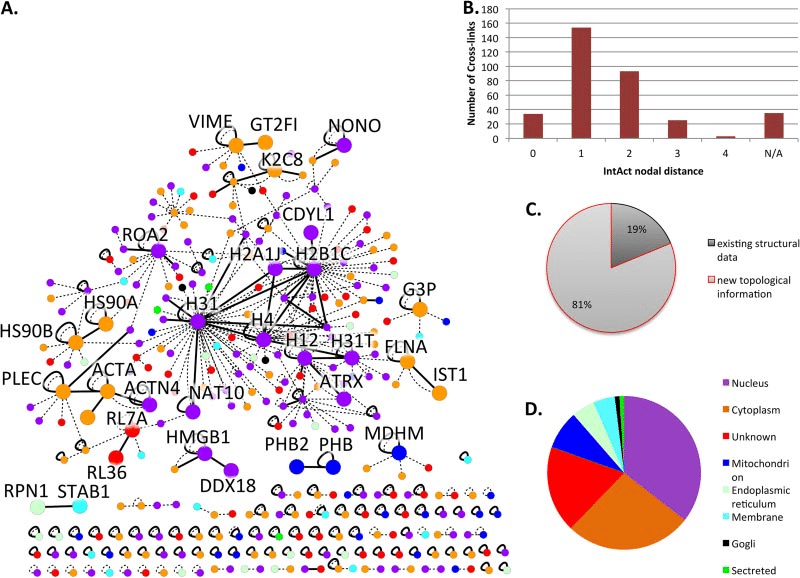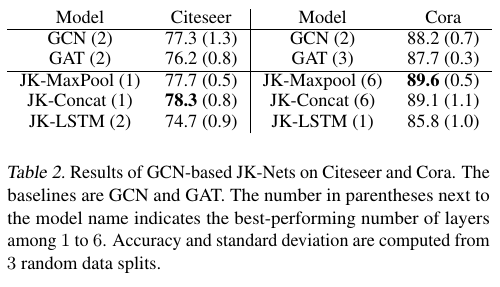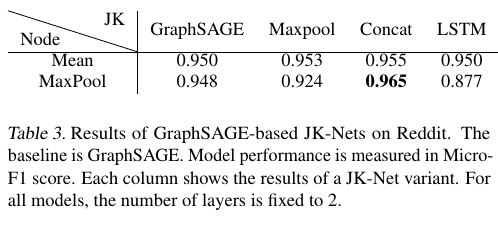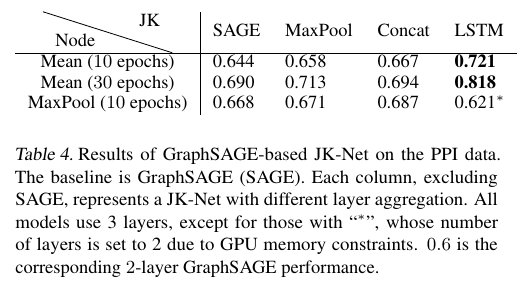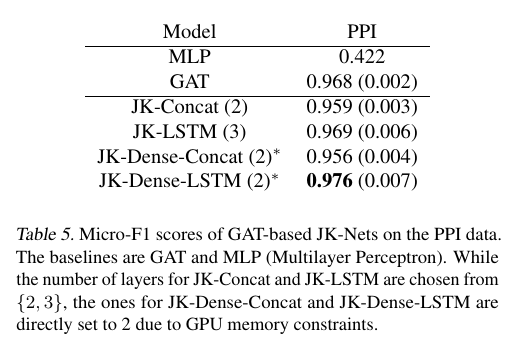# Related Work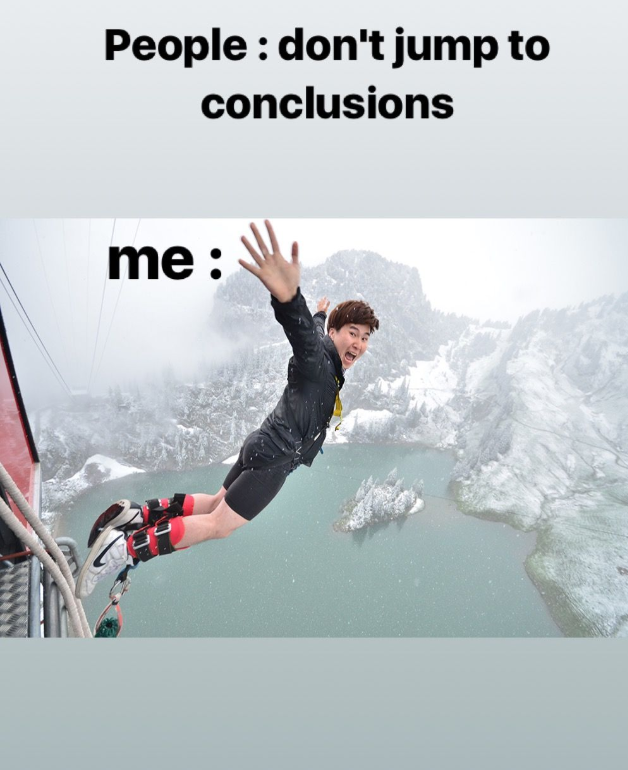Goal: Provide a representation learning scheme that can generalize better on diverse variety of network structure, than the one proposed for GCN's

Problem: Denser subgraphs may cause aggregation algorithms to converge in expectation to biased random walks. ☹

Solution: JK-Nets aggregate and leverage information from more than one hidden layers.😁

JK-Nets with the LSTM-attention aggregators outperform the non-adaptive models GraphSAGE, GAT and JK-Nets with concatenation aggregators.

### Future Work

Exploring other layer aggregators and studying the effect of the combination of various layer-wise and node-wise aggregators on different types of graph structures.

How can sequence modelling by itself impact the task of layer aggregation?

Are there smarter ways to keep track of node/community correlations within a network?

# ConclusionGoal: Provide a representation learning scheme that can generalize better on diverse variety of network structure, than the one proposed for GCN's

Problem: Denser subgraphs may cause aggregation algorithms to converge in expectation to biased random walks. ☹

Solution: JK-Nets aggregate and leverage information from more than one hidden layers.😁

JK-Nets with the LSTM-attention aggregators outperform the non-adaptive models GraphSAGE, GAT and JK-Nets with concatenation aggregators.

### Future Work

Exploring other layer aggregators and studying the effect of the combination of various layer-wise and node-wise aggregators on different types of graph structures.

How can sequence modelling by itself impact the task of layer aggregation?

Are there smarter ways to keep track of node/community correlations within a network?